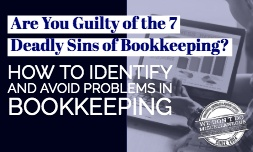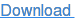#Depreciation is one of the hardest accounting practices to get right, but avoiding calculating depreciation could cost you money. Here's why you need to talk to your controller services about depreciation and what your options for claiming depreciation are.

## Are You Required to Claim Depreciation?

Many business owners who feel depreciation is too complicated or will require them to pay too much for an accountant consider not claiming depreciation. This is a mistake that could cost you far more than any savings from your accounting procedures.

## Claiming Large Asset Expenses

If you have an asset that will be used in your business for longer than the current year, you are generally not allowed to deduct its full cost in the year you bought it. Instead, you need to depreciate it over time. This rule applies whether you use cash or accrual-based accounting.

If you elect to not claim depreciation, you forgo the deduction for that asset purchase.

## Establishing a Cost-Basis for Asset Sales

When you sell an asset, you cannot make up for not taking a depreciation deduction by claiming a loss on the sale based on the original purchase price. You must use the depreciated value of the asset as your cost-basis whether or not you claimed depreciation expenses on your tax returns.

## Preparing Financial Statements

If you sell securities in your company and are required to follow Generally Accepted Accounting Principles, your income statement generally should reflect a depreciation expense and your balance sheet asset values should reflect the depreciated values. Even if you aren't required to follow GAAP, using depreciation better shows your company's true value and is of benefit both to you and potential investors.

## How to Choose a Depreciation Method

The purpose of depreciation is to give a rough estimate of an asset's current value and to spread its cost over the useful lifespan of the asset. There are three general categories of depreciation.

• Straight line depreciation spreads the cost evenly over a number of years.
• Accelerated depreciation writes off a greater portion of the cost in early years and a smaller portion in later years.
• Units of production depreciation writes off an asset as it is actually used.

## Straight Line Depreciation

Straight line depreciation is often chosen by default because it is the simplest depreciation method to apply. You take the asset's cost, subtract its expected salvage value, divide by the number of years it's expect to last, and deduct the same amount in each year.

For example, consider an \$11,000 asset with a \$1,000 salvage value that's expected to last 10 years. Subtract the \$1,000 in salvage value, divide the remaining \$10,000 by 10, and deduct \$1,000 in depreciation expenses each year for 10 years.

Straight line depreciation is properly used when an asset's value declines evenly over time. This would often be a piece of machinery that you expect to use until you scrap it.

## Accelerated Depreciation

Accelerated depreciation operates similarly to straight line depreciation, but instead of dividing evenly, early years are weighted more heavily. A common example is the double declining balance method.

To start, determine the depreciation rate by dividing 1 by the expected lifespan in years and then multiplying the result by 200 percent. In the above example, the depreciation rate would be 20 percent. That's double the depreciation rate in the straight line method.

• In the first year, you would deduct 20 percent of the asset's value (\$2,200).
• In the second year, you would take 20 percent of the remaining \$8,800 in value, for a \$1,760 deduction.
• You would continue this process each year until you have deducted a total of \$10,000 (\$11,000 original value minus \$1,000 in salvage value).

Accelerated depreciation is appropriate when an asset initially loses value quickly but then loses less value over time. The purchase of a new car is a good example. Other accelerated methods, such as the 1.5 balance method, may be used depending on how quickly an asset loses value.

## Units of Production Depreciation

The units of production method is used when an asset's lifespan is better calculated by how much it can produce rather than how long it will last. For example, a \$100,000 piece of production equipment might need to be disposed of after producing 1,000,000 units.

If you produce 300,000 units in the first year, you would use up to 30 percent of the asset's expected production. Therefore, you would deduct 30 percent of its original cost as a depreciation expense in that year (\$100,000 × 300,000/1,000,000 = \$30,000). In subsequent years, you would calculate that year's depreciation expense based on that year's production.

This method is most commonly used in industrial applications such as a die or mold that wears out as it's used.

## Modified Accelerated Cost Recovery System (MACRS)

The above methods are commonly used on financial statements, but for tax purposes, the IRS generally requires the use of MACRS. MACRS works similarly to accelerated depreciation.

The rate of depreciation and expected lifespan depend on the asset class. The IRS has tables in Publication 946 that give the appropriate depreciation rate and lifespan.

Note that certain low-value assets may be claimed as a one-time expense rather than depreciated over their expected lifespan if you desire. These are typically assets valued under \$2,500 if purchased by a small business or \$5,000 if purchased by a business required to file a 10-K. Check the IRS tables for the exact threshold and requirements.